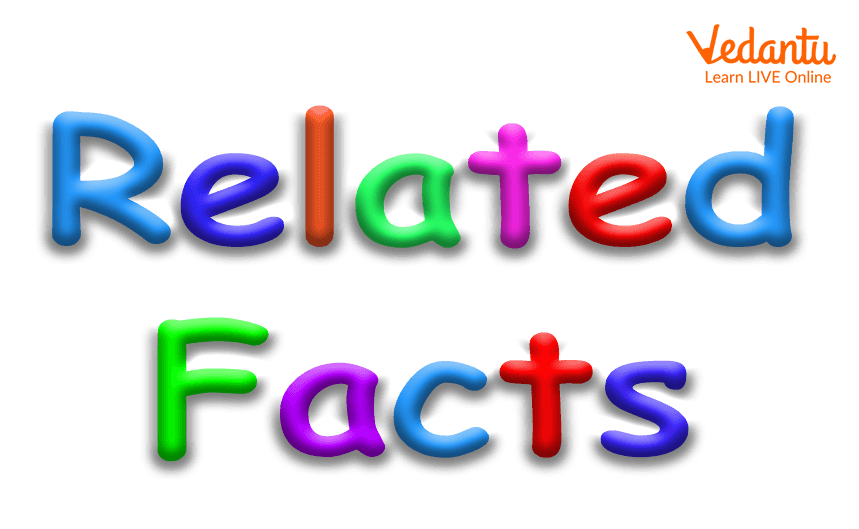Courses
Courses for Kids
Free study material
Free LIVE classes
More

# What are Related Facts?## Related Facts Examples

You have 5 chocolates. And, your best friend gives you 5 more. Now, how many chocolates do you have? Ten. Very Good! But, what if your sister asks you to give her 5 chocolates? Sharing is caring. Right? So, you give her 5 chocolates. Now, how many chocolates do you have? 5, right? Good.  That means if you add 5 with 5, you get 10 as a result. And, when you subtract 5 from 10, you will get 5. These are called related facts.Related Facts

## Related Facts

Related Facts are the Mathematical facts that derive the relationship between two different inverse operations such as addition and subtraction, and multiplication and division. These are the basic Mathematical expressions that are made up of three numbers.

## Related Facts of Addition and Subtraction

To understand the related facts of addition and subtraction, we will consider the following example:

4 + 6 = 10

6 + 4 = 10

10 - 4 = 6

10 - 6 = 4

Addition and subtraction are the inverse operations. In the above-given expressions, 4, 6, and 10 are the three numbers used. These numbers are related to each other using the ‘+’ or ‘-’ and ‘=’. In the first two examples, we perform the operation called addition. And, if we swap the numbers, we get the same result, that is 10. Also, in the last two equations, we subtract either 4 or 6 from the ‘10’, we get 6 or 4, respectively.

In addition and subtraction, related facts tell us that if we add one number, then subtract the same from the obtained result, they cancel each other.

For example, adding 6 with 4 gives the result of 10, and if we subtract 6 from 10, we will get 4. These types of facts are called the ‘related facts’. The point to be considered is that adding 6 with 4 gives the result of 10 whereas adding 4 with 6 gives the result of 10. This is called the commutative property of addition which is a ‘related fact’ of addition.

## Relationship of Multiplication and Division

Like addition and subtraction, multiplication and division are also inverse operations, which cancel each other.

To understand the related facts of multiplication and division, we will consider the following example:

6 x 2 = 12

2 x 6 = 12

12 ÷ 2 = 6

12 ÷ 6 = 2

A related fact about multiplication and division is that if we multiply a number by x (say), and then divide the result by x, then we will get the same number.

## Conclusion

By a given fact or a set of three numbers, we can write a total of four related facts. In addition, we can write three more facts using it. Similarly, we can write a total of four facts if a set of three numbers is of multiplication and division.

Last updated date: 20th Sep 2023
Total views: 137.4k
Views today: 1.37k

## FAQs on What are Related Facts?

1. How addition and subtraction are ‘related facts’?

Addition and subtraction are inverse in a relationship. They cancel each other. If we add a number and subtract the same from the result, then there will be no change in expression. Also, commutative property is considered a related fact. For example:

3 + 7 = 10

7 + 3 = 10

10 - 3 = 7

10 - 7 = 3

With any fact from the above given fact family, we can write its related three other facts simultaneously.

2. How multiplication and division are ‘related facts’?

Multiplication and division are related facts as they cancel each other and are also in inverse relationships. For example:

3 x 7 = 21

7 x 3 = 21

21 ÷ 7 = 3

21 ÷ 3 = 7

Multiplying by a number, and dividing the same number by the obtained result, causes no change in the expression. These facts are called related facts. Related facts are the basic Mathematical expressions consisting of three numbers.